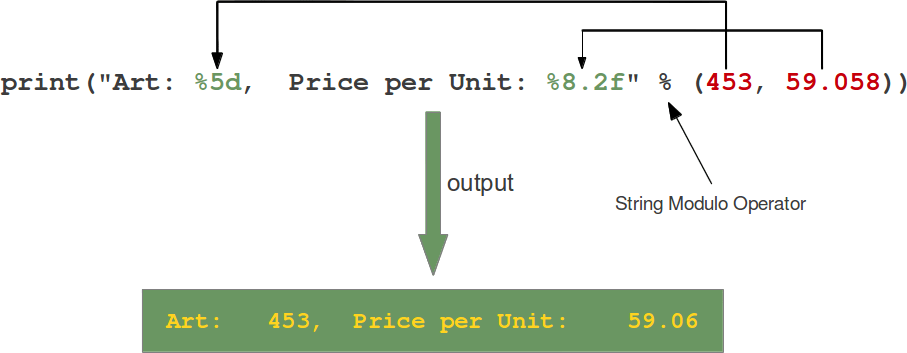# Quiz # 9

This week’s quiz consisted of creating a program to determine the distance between two points. You can check the mathematical way to do that here.

I did this quiz in two different ways. The first one was by defining a function with four different parameters -(x1, y1, x2,y2)- and asking the user for each value one by one:In the final output, I first had it displaying all the decimals in the answer. However, I didn’t like the way it looked, so I asked my teacher Ken how to make it so it only showed two decimals. We searched on the internet and found in this page the following image that shows how to do it:I did it, and this is how my program ran:The second way I in which I solved this quiz was by defining the function with only two parameters, each containing two values: the x and the y of the point. To do this, I used lists, like this:And here is the way it ran: